# Help me for this question by using the properties of regular language, please stven L= {oll p is prime? is not regular....

Help me for this question by using the properties of regular language, please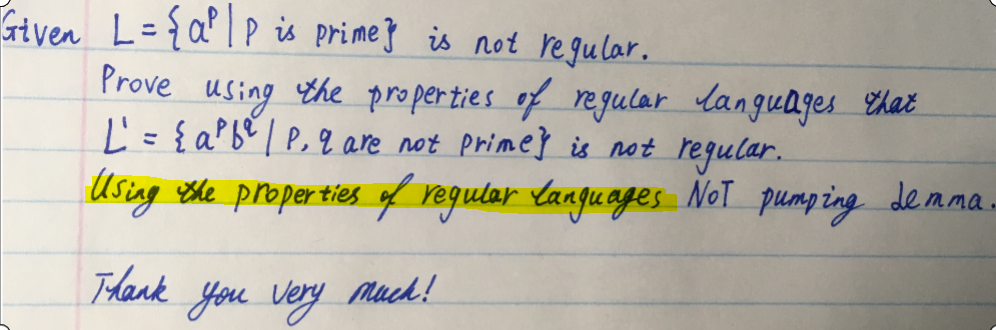stven L= {oll p is prime? is not regular. rove using the properties ofregu L' aIpl are not Prineinet egular. Usin, wepropertiestregudartangu呼es.NoT pumping-lemma FHank yuvery Muck!

Let L' = {apbq | p,q are not prime} be a regular language.

Then, by concatenation L' = L1.L2 where L1 = {ap | p is not prime} and L2 = {bq | q is not prime}
L1 and L2 are regular languages. (concatenation property)
L1' = {ap | p is prime} therefore L1' is also regular but this contradicts the given statement that {ap | p is prime} is not regular. (complement property)

Hence, our assumption that {apbq | p,q are not prime} is a regular language is also wrong.
Hence, proved.
Comment for any issues and upvote(like) if satisfied.

##### Add Answer of: Help me for this question by using the properties of regular language, please stven L= {oll p is prime? is not regular....
Similar Homework Help Questions
• ### 6.[15 points] Recall the pumping lemma for regular languages: Theorem: For every regular language L, there...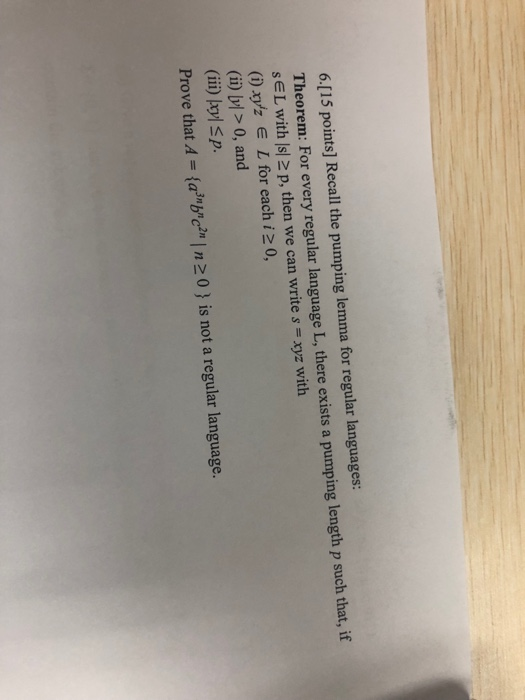6.[15 points] Recall the pumping lemma for regular languages: Theorem: For every regular language L, there exists a pumping length p such that, if s€Lwith s 2 p, then we can write s xyz with (i) xy'z E L for each i 2 0, (ii) ly > 0, and (iii) kyl Sp. Prove that A ={a3"b"c?" | n 2 0 } is not a regular language. S= 6.[15 points] Recall the pumping lemma for regular languages: Theorem: For every regular...

• ### 4. (15 points) Using the pumping lemma for regular languages show that the following language is...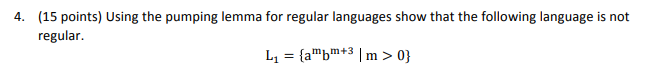4. (15 points) Using the pumping lemma for regular languages show that the following language is not regular

• ### Can someone use pumping Lemma to show if these are regular languages or not c) Is...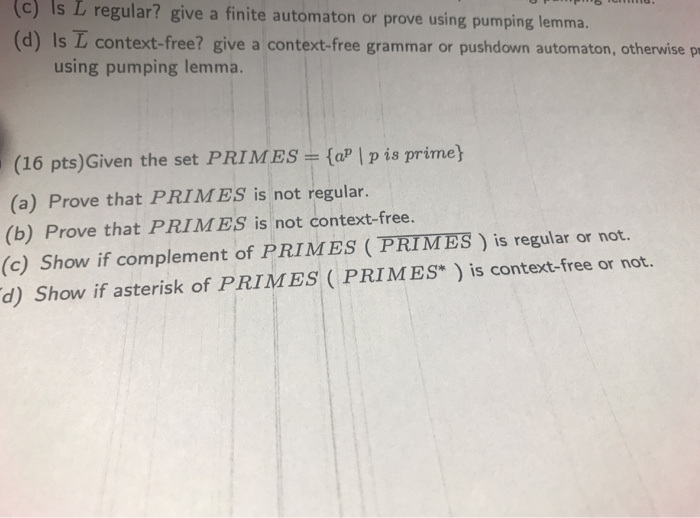Can someone use pumping Lemma to show if these are regular languages or not c) Is L regular? give a finite automaton or prove using pumping lemma. (d) Is L context-free? give a context-free grammar or pushdown automaton, otherwise pr using pumping lemma. (16 pts)Given the set PRIMES (aP | p is prime (a) Prove that PRIMES is not regular. (b) Prove that PRIMES is not context-free. (c) Show if complement of PRIMES (PRIMES ) is regular or not. d)...

• ### 6. (10 pts) Is L regular? Either prove that it is not regular using pumping lemma,...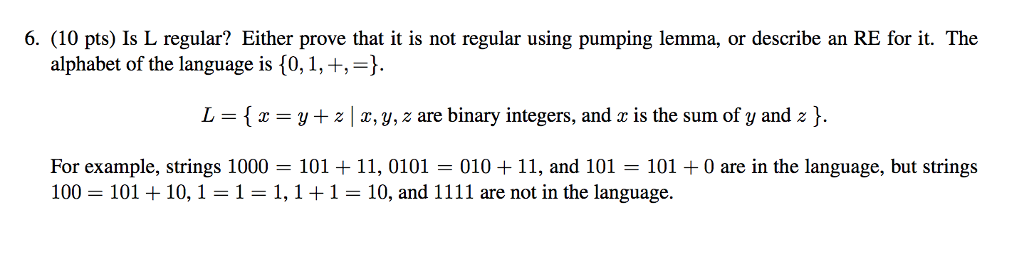6. (10 pts) Is L regular? Either prove that it is not regular using pumping lemma, or describe an RE for it. The alphabet of the language is 10,1, +,-) L = { x = y + z | x, y, z are binary integers, and x is the sum of y and z }. For example, strings 1000 = 101 + 11, 0101 = 010 + 11, and 101 = 101 + 0 are in the language, but strings...

• ### Prove that the language L = {a^pb^qc^r|p,q,r >=1 and pq = r} is not context free using pumping lemma. I honestly just...

Prove that the language L = {a^pb^qc^r|p,q,r >=1 and pq = r} is not context free using pumping lemma. I honestly just need help coming up with a string that works, thanks.

• ### Pumping lemma proof by contrapositive

Please Help me complete the following proof. I've done some of the set up but not quite sure where to go from where I am. First of all I'm not sure what to pickfor s. After that I think I may be able to figure it out if you wish to stop there. Thanks for any help you can provide.Consider the language L = {aibjcj where i>=1 and j>= 0} . Prove that this language is non-regular using thecontrapositive of...

• ### State whether or not it is regular.

Question Detailsstate whether or not it is regular.(1) All strings over the alphabet {a,b,d,l,r} that contain at least one of the words bad, bald, ball or bard.If you claim the language is not regular, prove your claim using the Pumping Lemma.If you claim the language is regular,– Give a regular expression that matches exactly the strings in the language,– Give a graphical representation of an NFA that recognizes the language,– Convert the NFA into a DFA that recognizes the language,...

• ### SUBJECT:THEORY OF COMPUTATION CAN SOMEONE PLEASE HELP ME I HAVE POSTED IT REPEATEDLY AND I KEEP G...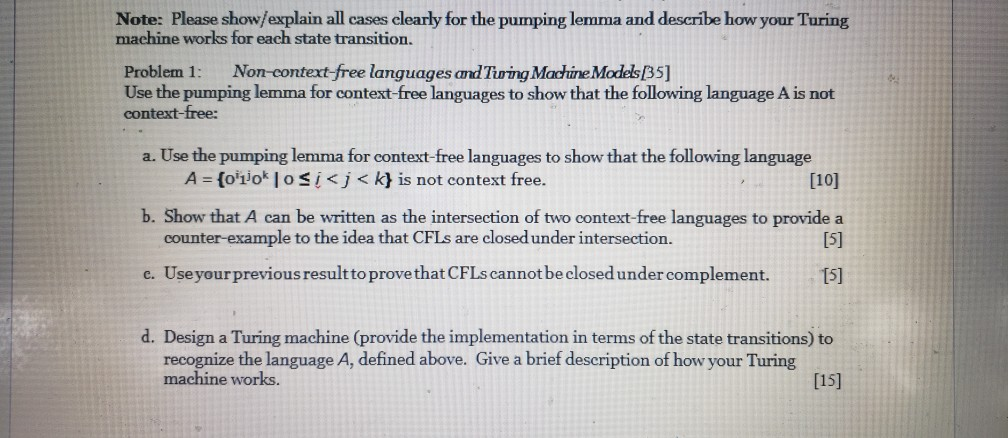SUBJECT:THEORY OF COMPUTATION CAN SOMEONE PLEASE HELP ME I HAVE POSTED IT REPEATEDLY AND I KEEP GEETING INCOMPLETE / INCORRECT ANSWER . I WILL GIVE YOU A HIGH REVIEW IF YOU HELP ME AND IT IS DONE PROPERLY ! Note: Please show/explain all cases clearly for the pumping lemma and describe how your Turing machine works for each state transition. Problem 1: Non-context-free languages and Tining Machine Models B5] context-free: 쉑: Use the pumping lemma for context-free languages to show...

• ### (Automata): prove using the pumping lemma that the following language is not context-free: where: ; b)using...

(Automata): prove using the pumping lemma that the following language is not context-free: where: ; b)using closure properties and the previous proof, show that the following language is not context free language: Really need your help with this, it is important for the test. please explain what you to do so i can study it throughly. thank you very much! Labc be...bc2m de fefefnghqhq.h 1, т > п> о >0; > т,п, о 0; /12, ...j2n0; k1, k2,.. k, >...

• ### 6. |10 Points) Let L be a language over Σ {a.b}. Using L, we define a new language double(L) which includes the strings u u . for every string u in L. Show that double (L) is regular whenever L is re...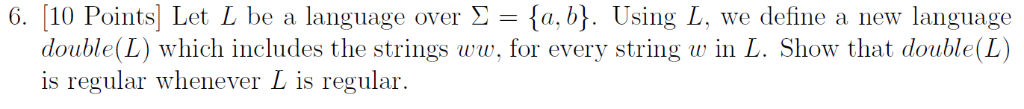6. |10 Points) Let L be a language over Σ {a.b}. Using L, we define a new language double(L) which includes the strings u u . for every string u in L. Show that double (L) is regular whenever L is regular 6. |10 Points) Let L be a language over Σ {a.b}. Using L, we define a new language double(L) which includes the strings u u . for every string u in L. Show that double (L) is regular...

Need Online Homework Help?